## Thursday, July 26, 2012

### Crash Course on Notation in Programming Language Theory

This blog post is meant to help my friends get started in reading my other blog posts, that is, this post is a crash course on the notation used in programming language theory ("PL theory" for short). For a much more thorough introduction, I recommend Types and Programming Languages by Benjamin C. Pierce and Semantic Engineering with PLT Redex by Felleisen, Findler, and Flatt. I'll assume the reader is an experienced programmer but not an experienced mathematician or PL theorist. I'll start with the most basic definitions and try to build up quickly.

### Sets, Tuples, Relations, and Definition by Rules

I suspect many readers will already be familiar with sets, tuples, and relations and thus may want to skip the next few subsections, but if you are not familiar with inductive definitions, then please make sure to read the subsection below titled Definition by Rules.

#### Sets

The main building block that we use in PL theory is the set, a collection of objects (also called elements), such as the set containing the first three natural numbers: The only thing that matters is whether an object is in the set or not; it doesn't make sense to ask if there are duplicates of an object or in what order the objects appear in the set. For example, the set $\{ 0,2,1 \}$ is the same set as the set listed above. The notation $\in$ means "in", so $1 \in \{ 0,1,2\}$ is true and $3 \in \{ 0,1,2\}$ is false. Sets may have an infinite number of elements, such as the set of all natural numbers (non-negative integers), written $\mathbb{N}$.

#### Tuples

Another building block is the tuple, which is an ordered collection of objects. So $(0,1,2)$ is a tuple of three elements and it is different from the tuple $(0,2,1)$. The subscript notation $t_i$ retrieves the ith element of tuple $t$. For example, if $t=(0,2,1)$, then $t_1=0,t_2=2,t_3=1$. Tuples contain only a finite number of elements and usually less than a handful. Sometimes angle brackets are used for tuples instead of parentheses, such as $\langle 0,2,1 \rangle$.

#### Relations

Putting tuples and sets together we get relations. That is, a relation is a set of tuples. We often use relations to represent a mapping from input to output. For example, the above relation can be thought of as mapping a natural number to its successor, that is, to the next greater natural number. The above definition is rather imprecise because of the elipses ($\ldots$). Fortunately, there are more precise notations for describing infinite sets and relations.

#### Definition by Rules

The main way that we define infinite sets in PL theory is by giving a list of rules for what is in the set. Let's use the name $R$ for the above relation. Then the following two rules give a precise definition of $R$. Notice that the second rule is recursive in that it refers to itself. That's ok and quite common.
1. $(0,1) \in R$.
2. For any natural numbers $n$ and $m$, if $(n,m) \in R$, then $(n+1,m+1) \in R$.
When we use rules to define a set, we implicitly mean that an element is not in the set if there is no way to use the given rules to justify that the element should be in the set. So $(0,0)$ is not in $R$ because there is no way to use the above two rules to conclude that $(0,0)$ is in $R$.
Some sets of rules are nonsensical and do not define a set. For example, rules should not be contradictory as in the following.
• $(0,1) \in R$.
• $(0,1) \notin R$.
A textbook on set theory will give the restrictions on what constitutes a "good" bunch of rules, but we won't go into that here, other than to point out that you need at least one non-recursive rule and that logical negation should be avoided.
A common notation for rules such as the above uses a horizontal line in place of "if" and "then". For example, an equivalent definition of $R$ is given by the following. We have dropped the "For any natural numbers $n$ and $m$" part of rule 2. The convention is that variables such as $n$ and $m$ that appear in a rule can be replaced by any object of the correct type, in this case, a natural number. Often the "correct type" is something you can deduce from the context of the discussion, in this case, the natural numbers.
Suppose that I claim that a particular element is in $R$, say $(2,3) \in R$. You might respond by saying that you don't believe me. To convince you, I need to show you how the rules justify that $(2,3) \in R$, that is, I need to show you a derivation. A derivation is a chaining together of rules, replacing variables such as $n$ and $m$ with particular objects and replacing premises such as $(n,m) \in R$ with sub-derivations. I've labelled each step in the derivation with the rule number.
The fancy name for what I'm calling Definition by Rules is inductive definition. (My daughter love the Fancy Nancy series of books.)

### Language Syntax and Grammars

It turns out that using rules to defines sets, as we did above, is how we define the syntax of a programming language. Suppose we'd like to define a simple language of integer arithmetic, call it $\mathit{Arith}$, including expressions such as 1 + 3 and -(5 + 2). Recall that $\mathbb{Z}$ is the set of all integers. Then here's a bunch of rules that we might use to define $\mathit{Arith}$:
• For any $i\in \mathbb{Z}$, $i \in \mathit{Arith}$.
• For any $e$, if $e \in \mathit{Arith}$, then -$e \in \mathit{Arith}$.
• For any $e_1$ and $e_2$, if $e_1 \in \mathit{Arith}$ and $e_2 \in \mathit{Arith}$, then $e_1\mathtt{+}e_2 \in \mathit{Arith}$.
• For any $e$, if $e \in \mathit{Arith}$, then $\mathtt{(}e\mathtt{)}\in \mathit{Arith}$.

Backus-Naur Form (BNF) is another common notation for writing rules that define the syntax of a language, but the meaning is the same. (There are several variations on BNF; I forget which one I'm using here.) The bunch of rules is referred to as a grammar.
Arith ::= integer
Arith ::= "-" Arith
Arith ::= Arith "+" Arith
Arith ::= "(" Arith ")"

A vertical bar (meaning "or") is often used to make such syntax definitions more concise, as follows.
Arith ::= integer | "-" Arith | Arith "+" Arith | "(" Arith ")"

In PL theory, we use a peculiar variation on BNF that replaces the name of the language being defined, in this case $\mathit{Arith}$, with the variable that is used to range over elements of $\mathit{Arith}$. So suppose we are using the variable $i$ as a placeholder for any integer and $e$ as a placeholder for elements of $\mathit{Arith}$. Then we would write the above grammar as Note that I've dropped the parentheses. It's generally understood that parentheses are allowed in any language.
The notion of derivation coincides with that of a parse tree, they both demonstrate why a particular element is in a set.

### Operational Semantics

A language is brought to life by defining what it means to run a program in the language, that is, the operational semantics of a language. In the case of $\mathit{Arith}$, we just need to specify the integer output of each program. As discussed above, relations can be used to map inputs to outputs, and indeed, we typically use relations for this purpose in PL theory. There's several different styles of relations, the first we'll discuss is a big-step style of semantics that maps a program directly to its output.

#### Big-step Semantics

Let's define a relation $\mathit{Eval}$ that maps elements of $\mathit{Arith}$ to integers. For example, we'd like to have $(\mathtt{-(2 + -5)},3) \in \mathit{Eval}$. This relation will be infinite (because there are an infinite number of programs in $\mathit{Arith}$), so again we'll use a bunch of rules to define $\mathit{Eval}$. But before we start, it's common to introduce some shorthand: $e \Downarrow i$ means $(e,i) \in \mathit{Eval}$. Below we state the rules that define $\mathit{Eval}$ using the horizontal notation. To make sure we don't leave out any programs, we create one rule for each syntactic rule of $\mathit{Arith}$ (there are three). We say that the rules are syntax-directed when there is one rule for each syntactic rule in the language. It may seem a little odd that I'm defining - in terms of $-$, and similarly for +. Isn't that circular? No, the $-$ and $+$ are the usual arithmetic operators for integers that everyone learned in grade school. In this way, the $\mathit{Arith}$ language is rather odd in not using 32 or 64-bit arithmetic. An implementor of $\mathit{Arith}$ would have to use a big-integer package to properly handle the arithmetic.

#### Small-step Semantics

The second, and perhaps more common, style of operational semantics is small-step semantics. In this style, the relation doesn't map a program to its output, but instead it maps a program to a slightly simplified program in which one subexpression has been computed. This style of semantics can be thought of as textual rewriting. To give an example of this style, let's define a relation named $\mathit{Step}$. We'll want this relation to have the following elements, among many others: Again, we'll introduce shorthand: $e_1 \longrightarrow e_2$ means $(e_1,e_2) \in \mathit{Step}$. Also, we'll chain together steps, so $e_1 \longrightarrow e_2 \longrightarrow e_3$ means $e_1 \longrightarrow e_2$ and $e_2 \longrightarrow e_3$. The term reduce is a synonym for step. The above example of two steps can now be written as OK, on to the rules that define the $\mathit{Step}$ relation. There are five rules, which we explain below. Rules (1) and (2) are the most interesting; they perform the arithmetic. We call them computational reduction rules. Rules (3-5) allow us to reach inside of sub-expressions to perform computation. They are often called congruence rules for reasons we won't go into. The use of the variable $i$ in rule (5) means that reduction proceeds from left to right. In particular, we're not allowed to reduce the right-hand expression of a plus until we've already reduced the left-hand side to an integer.
Aside: This left-to-right ordering is a choice that I made as the designer of this example language. I could have not specified an ordering, letting it be non-deterministic. However, this example language doesn't have side-effects, so the ordering doesn't matter! However, most language do have side-effects and they do specify an ordering (but not all!), so I thought to include an example of how ordering is typically specified.
Time for an example: let's see the derivation of the step $\mathtt{-(2 + -5)}\;\longrightarrow\; \mathtt{-(-3)}$.
We've defined a single step of computation, the $\mathit{Step}$ relation, but we're not quite done. We still need to specify what it means to run a program to completion. We'll do this by defining a relation $\mathit{Eval}'$ in terms of the $\mathit{Step}$ relation as follows. In plain Engilish, the $\mathit{Eval}'$ relation will contain any pair $(e,i)$ if expression $e$ reduces to integer $i$ in zero or more steps. Some of the notation here is new and is explained below. The notation $\{ \ldots \mid \ldots \}$ is the set builder or set comprehension notation for defining a set. The stuff to the left of the vertical bar is a template for a typical element of the set and the stuff to the right of the vertical bar places restrictions on the elements in the set. The notation $\longrightarrow^{*}$ means zero or more steps. I like to define this multi-step relation with the following rules: (My brain is good at reasoning about Lisp-style lists, so I think of the first rule as nil and the second rule as cons.)

### Type Systems (with the Lambda Calculus as an example)

Many programming languages are statically typed, that is, the compiler performs some sanity checking before proceeding with the actual work of compiling. The checking usually involves make sure that objects are only used as intended, for example, not trying to treat an integer as if it were a function. The way a programming language designer (PL theorist) specifies what kind of sanity checking should be performed is by defining a type system for the language. The $\mathit{Arith}$ language is so simple that there is no interesting type checking to be performed. Let's consider a slightly larger language that also happens to be used over and over again in PL theory, the lambda calculus (technically, the simply-typed lambda calculus). The lambda calculus just consists of first-class anonymous functions. Here we'll extend the lambda calculus to also include our arithmetic expressions. So now our example language is defined by the following grammar. The $x$ variable ranges over parameter names, such as foo and g. Two expressions right next to each other denote function application (i.e., function call). So if you're familiar with the C language, read $e_1 \, e_2$ as $e_1(e_2)$. In the lambda calculus, functions only take one parameter, so function calls only require one argument. The syntax $\lambda x{:}T.e$ creates a function with one parameter named $x$ of type $T$ (types will be defined shortly) and whose body is the expression $e$. (A common point of confusion is to think that $x$ is the name of the function. It instead is the parameter name. The function is anonymous, i.e. it doesn't have a name.) The return value of the function will be whatever the expression $e$ evaluates to.
Now let's consider what kind of objects will be alive when we run the program: there's integers and functions. We'll create a set of types to describe the kinds of objects, using $T$ to range over the set of types. In a function type $T_1 \to T_2$, the $T_1$ is the type of the parameter and $T_2$ is the return type.
The job of a type system is to predict which type of value will be produced by an expression. For example, the expression -(5 + 2) should have the type Int because the result of -(5 + 2) is -3, which is an integer. As with the syntax and operational semantics of a language, PL theorists use relations and rules to define a type system. We'll define a relation named $\mathit{WellTyped}$ that, as a first approximation, maps expressions to types, so for example, we'll have $(\mathtt{-(5 + 2)},\mathtt{Int}) \in \mathit{WellTyped}$.
However, because the lambda calculus includes variables, we'll need something analogous to a symbol table, a relation called a type environment, to keep track of which variables have which types. The Greek letter $\Gamma$ (gamma) is traditionally used for this purpose. We'll need to be able to create new type environments from old ones, potentially overshadowing variable definitions from outer scopes. To set up the mathematical machinery for that, we define $\Gamma \backslash x$ to be the relation just like $\Gamma$ except that any tuple starting with $x$ is removed. (The way the type system will be defined, there may be 0 or 1 tuple that starts with $x$, making the type environment a special kind of relation called a partial function.) We'll write $\Gamma,x{:}T$ for the operation of extending a type environment with variable x, possibly overriding a previous definition, and define it as follows: Suppose we have Then
One way in which type environments are different from the global symbol table in a compiler is that there isn't just one type environment, there will be lots of them, one for each scope. Further, we won't ever update a type environment in place, we'll keep creating new ones that differ a little bit from the old ones. From a programming perspective, the mathematical metalanguage we're using here is pure functional, that is, it doesn't use state or side effects. The reader might worry that this might lead to inefficiency, but remember, we're not writing a program here, we're writing a specification! Clarity is what matters most in this setting, and staying pure helps to make things clear.
Getting back to the $\mathit{WellTyped}$ relation, instead of containing 2-tuples (pairs) it will contain 3-tuples (triples) of the form $(\Gamma,e,T)$, so we'll be assigning types to expressions in the context of a type environment. As yet more shorthand (PL theorists love shorthand!), we'll write $\Gamma \vdash e : T$ instead of $(\Gamma,e,T) \in \mathit{WellTyped}$. We're now ready to write down the rules that define $\mathit{WellTyped}$. To sum up the above rules, the arithmetic operators work on integers, variables get their types from the environment, lambdas are given function types based on their parameter type and their deduced return type, the body of a lambda is checked using the environment from the point of creation (this is lexical scoping) extended with the lambda's parameter, and function application is sane so long as the argument's type is the same as the parameter type.

### Conclusion

Thus ends this crash course on the notation used in programming language theory. This blog post only scratches the surface, but much of the additional notation that you'll need is variations on what's covered here. Happy reading! And P.S., feel free to ask questions in the form of comments to this blog.

#### 39 comments:

1.Anonymous1:13 PM

Excellent idea. I was about to ask you for a cheat sheet for your previous blog. I studied operational semantics but, since I'm not using it day to day, I needed a refresher. One question: is the rho in your previous post the same as capital gamma here?

1.Hi Bartosz, the rho is related but different from the gamma. The rho is a runtime environment that maps variables to values whereas gamma is a type environment, mapping variables to types. The two should be related in that the value for a given variable should have the type given by the type environment.

2.Anonymous2:15 PM

That makes sense: ρ plays the role of the heap.

There is one more piece of notation that's needed to understand your previous blog: the angle brackets in:

lt;λx.e, ρ>

(It seems like I can't embed any tags in a comment)
My understanding is that the big-step value of a lambda is a closure, which is the lambda paired with the captured environment.

3.It's not quite right to think of rho as the heap. It's a bit closer to the procedure call stack, because it stores the values for function parameters, but that's also not quite right because it includes variables from enclosing scopes. You might read my post about closure conversion, which talks about translating from the high-level notion of a first-class function with lexical scoping, down to the lower-level notion of functions without lexical scoping.

Yes, the angle brackets are just another notation for pairs.

2.> “In PL theory, we use a peculiar variation on BNF that replaces the name of the language being defined, in this case Arith, with the variable that is used to range over elements of Arith. So suppose we like to use the variable i as a placeholder for any integer and e as a placeholder for elements of Arith.”

The significance of this is lost on me. It looks like you're simply replacing one name with another. Is there something deeper going on here?

1.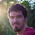I think the difference is that the set (Arith or integer) is replaced by its general element (e or i) in the new definition.

2.Hi Dave, you're right, there's nothing deep going on there.

3.Stray bit of LaTeX on this page I think (Search for mathtt in your browser).

1.Also, Backus-Naur instead of Backus-Nauer and there is a consistent error in the examples of small-step sematics (-(-3) -> -3 which should be 3)

2.Thanks! Fixed.

4.“The use of the variable i in rule (5) means that reduction proceeds from left to right. In particular, we're not allowed to reduce the right-hand expression of a plus until we've already reduced the left-hand side to an integer.”

I'm also wondering why this is important. Presumably you can prove things using these rules even if the order of computation is nondeterministic. But then, I guess… you also end up having to prove that the system of rules is consistent and produces the same result when evaluated in any order?

1.As the designer of the example language, I could have left the order unspecified, making it non-deterministic. However, for the
example language, it doesn't matter because it doesn't have
side-effects. As you point out, one could prove that it doesn't
matter, that the language is confluent. Of course some languages have side-effects and non-deterministic, so reduction is those languages is not confluent.

I decided on left-to-right ordering for this example because its quite common and I wanted to show readers how that is typically achieved.

5.I found the paragraph where you introduce Γ (gamma) to require too much imagination on my part to read it last night, and felt like you lost me just before the big payoff. Deconstructing…

> Actually, because the lambda calculus includes variables, we'll need something analogous to a symbol table, a relation called an environment, to keep track of which variables have which types. The Greek letter Γ (gamma) is traditionally used for this purpose.

Okay so far, but now I have to guess how this relation works. My first guess would be that it maps symbol names to types... but then there's the whole problem of scope, since one lambda can call another and presumably can use the same parameter name. So, examples would be a big help here.

> We'll write Γ∖x for the relation just like Γ except that any tuples starting with x are removed.

Okay, but what's the significance of this? It would help if you'd tell us what you're trying to do with Γ∖x before we start to talk about it.

Also, “any tuples starting with x are removed” seems to imply that x can have multiple types in Γ. Is that really what you mean, or should it be “any tuple starting with x is removed?” (I note you never said whether relations can be one-to-many or not).

> We'll write Γ,x:T as shorthand for (Γ∖x)∪{(x,T)}.

So, in English: “Γ,x:T is the symbol table Γ, except that the type of x is T, replacing any existing type for x if necessary.” Did I get that right?

> So getting back to our example, we'll have (∅,−(��+��),������)∈WellTyped. As yet more shorthand (PL theorists love shorthand!), we'll write Γ⊢e:T instead of (Γ,e,T)∈WellTyped.

Whoa; what does it mean to have a triple in WellTyped? I understood from “WellTyped that maps expressions to types, so for example, we'll have (−(��+��),������)∈WellTyped” that it would contain pairs. Are there parens missing here?

I would consider saying less about sets (doesn't everyone learn about sets by the time they're in high school?) and doing more to elaborate this section.

1.Thanks for the feedback Dave! I've updated the text to clarify and answer your questions. But to answer your direct questions:

Yes, you understand type environment extension (Gamma,x:T)
correctly.

No missing parens, the WellTyped relation is really over triples.

6.Excellent post and great content overall. I would like to ask you something Jeremy.

Can a relation (--(5+2), 7) be reduced by your rules? Should we reduce the relation (e,i) simultaneously (and conclude that it cannot be reduced with these rules only) or should we start with the --(5+2) and in three steps ((2)->(3)->(3)) we conclude to 7?

I believe that this relation cannot be reduced, but I still feel the need to ask.

Thank you,
Aggelos

1.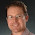Dear Aggelos,

Thank you.

Are you asking whether the pair (--(5+2), 7)
is in the relation -->*? or whether it is
in the relation Eval? The answer either way is yes.
The following shows that --(5+2) -->* 7.

--(5+2) --> --7 --> 7

For the big step version (but writing the downarrow as =>), we have

5 => 5 2 => 2
------------------
5 + 2 => 7
-----------------
-(5 + 2) => -7
-----------------
--(5 + 2) => 7

Cheers,
Jeremy

2.Of course! I was confused with big step. I was evaluating the --(5+2) to the final result 7 and erroneously trying to find a rule in your small step semantics to reduce it right away, like you did in the big step version.

Thank you very much.

7.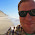Thank you very much for this post! This info is what finally got me through the notation soup in a microsoft research paper on serializing closures in cloud haskell.

1.You're very welcome!

8.Anonymous8:57 AM

Is zero (0) in the set of N?

1.Yes. (Not everyone agrees that zero is a natural number, but PL theorists generally include zero as a natural number.)

9.I wish I had had this page when I was studying for my comprehensive exam on PL and type theory. This would have saved a lot of time and unnecessary confusion. Unfortunately a lot of math books assume that everyone knows the notations, or they define the principle notations but not all the details, so articles like this are very useful.

10.Thanks for this post! It's very helpful! (But note that lots of stuff disappears if Javascript is disabled.) I recommend amending your introduction to Γ (type environments) with an example so that the remaining 2/3 of the introductory paragraph is easier to understand. I mean, it says "just like Γ" but the reader does not yet have a clear idea of what Γ is.

The way small-step semantics works seems weird to me. Although there is a set of rules, it is not necessarily obvious how to apply them. That is, given the rules I suspect it is non-obvious in general how to write a compiler to apply them to an arbitrary input (and it could be especially difficult if the reader does not understand the meaning or purpose of the rules, which tends to happen in these terse PL papers, right?) The example language "e ::= i ∣ −e ∣ e+e" is simple enough that one can figure out how to apply the rules; although I fully understood the language from the start, yet I had to squint my eyes and scratch my head to figure out what was going on with the rules. Oh well, maybe with practice it gets easier. You've shown the derivation of −(2+−5) -> 3, but in general we begin with the input only, and have to determine the output based on the rules. That seems harder... especially if you're not sure what the paper is trying to accomplish. It's not clear to me if the "Eval" relation helps with this problem, maybe you could add a concrete example of an Eval relation?

Now here's a paragraph that should be added to half the PL papers in existence:

"The x variable ranges over parameter names, such as foo and g. Two expressions right next to each other denote function application (i.e., function call). So if you're familiar with the C language, read e1e2 as e1(e2). In the lambda calculus, functions only take one parameter, so function calls only require one argument. The syntax λx:T.e creates a function with one parameter named x of type T (types will be defined shortly) and whose body is the expression e. (A common point of confusion is to think that x is the name of the function. It instead is the parameter name. The function is anonymous, i.e. it doesn't have a name.) The return value of the function will be whatever the expression e evaluates to."

Most papers take big shortcuts, failing to define what "x", "e", etc. mean. Apparently the variables names are supposed to be self-explanatory ("oh, we don't have to say that e is an expression, everyone knows that automatically!") If only PL papers included a link to this blog post, it wouldn't be so bad. But they don't.

Another shortcut they'll often take is to define a bunch of operators like have a short definition like "e::= e∆e | e₰e | e e | ..." but with no precedence rules, and then they expect you to know what "a ∆ b c ₰ d" means (even this post uses that shortcut, leaving us to wonder whether "Γ ⊢ e : T" means "Γ ⊢ (e : T)" or "(Γ ⊢ e) : T" (perhaps it is supposed to be obvious, but it's not because there is no independent definition provided for "⊢" and ":"). Sometimes I can't even figure out if some symbol ₹ is a variable or an operator... argh! Even phrases as simple as "T → T" can leave puzzles in the reader's mind, like "oh, does this mean A → A legal but A → B is illegal?"

I mean, is there something wrong with me for thinking that papers should be written such that any programmer who has enough skills to write a C compiler can understand them?

Conclusion: ranting about PL papers is a lot more fun than reading them. But thanks to this blog post, the job just got easier! Uhhh... of course, those poor souls seaching Google for things like ⊢ and Γ will not actually be able to find this blog post ("your search - ⊢ - did not match any documents.") Oh well.

1."I mean, is there something wrong with me for thinking that papers should be written such that any programmer who has enough skills to write a C compiler can understand them?"

I don't think this is reasonable; it's rather like saying that papers on quantum mechanics should be readable by anyone who can build a particle accelerator. They're related but not the same. You can write a C compiler and not be conversant with PL theory and terminology. Many of the things you cite as confusing are common knowledge and conventions among computer scientists and mathematicians who study programming languages; it would be very repetitious to include basic explanations and definitions of the field in every paper. Imagine if someone said that every program written in C should be preceded by a set of comments explaining the syntax and semantics of C...

11.Feel free to write more of these introductory posts; I'm more of a compiler guy, and I often get lost when I try to read something about PLT.

12.Thank you for this post! Notations have kept me from reading quite a few papers over the past few months! It's a breath of fresh air to know that nothing in this post went over my head.

13.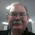Thanks. I'm reading Types and Programming Languages right now. You're discussing relations then types with the Lambda Calculus. How does the Relational Algebra or Relational Calculus underpinning the relational data model fit into this?

14.Anonymous12:58 AM

This is great, though there was one bit that is much less obvious to me than all the rest.. " the body of a lambda is checked using the environment from the point of creation (this is lexical scoping) extended with the lambda's parameter". I'm going to think about it some more, as a good exercise.

In the example where you demonstrated left-to-right ordering I could easily figure out what the alternative would be, but I don't see how you could write this differently and get a different type of scoping.

15.Anonymous3:04 AM

Minor spelling error:

"sytnax-directed" probably should be "syntax-directed" :-)

1.Thanks, fixed!

2.While you're there :-)

Calclus -> Calculus

3.Thanks, fixed that too!

16.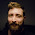Thank you for this post. It cleared many things in my head - my mind kept saying 'proves' for ⊢ before. Is there any relationship with a proof, or is this just 'notational coincidence'?

I recently got Pierce's TAPL and am working through the first chapters. Are congruence and confluence touched in the text, or should I look for it somewhere else?

1.Yes, a derivation is a proof.

Congruence rules as I discuss above are in TAPL; rules like that appear in every language.
Confluence is only briefly discussed in TAPL.

17.Great post, worth bookmarking. I often get headache with this symbols now I have some place to look, good job.

18.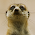Hi Jeremy,

Thanks this was a fantastic introduction!

Do you have any advice for further reading following on from this post? (Blogs, books etc?)

Would you recommend "Essentials of programming languages" or "Types and programming languages" as the next step?

Thanks again

1.Both EOPL and TAPL are great books, but they are very different. EOPL is about understanding programming languages by creating interpreters for them. TAPL is about formalizing the semantics of languages and proving properties about them.

19.Thanks, this is a big help.

But, what does an overline mean, I know of about 5 different meanings for it, i.e.

\left{ \overline{l:x} \right}

Also, what do the squiggly arrow, exclamation mark, and at sign mean.

thanks much

1.Where do you see that notation being used? I don't recall using it in this blog post... are you referring to some paper?